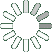Puzzle Command
Daily Puzzle
Number Logic #16
February 15, 2015Find a 6 digit number where:

The 1st digit is equal to 1.
The 2nd digit is equal to 2.
The 3rd digit is equal to 3.
The 4th digit is the sum of the 1st, 2nd, and 3rd digit.
The 5th digit is the total count of 1s in the number.
The 6th digit is odd and not equal to 8 or 9.

Puzzle Notes
6 digit numbers range from 100,000 to 999,999.
Enter in your solution below and click submit.